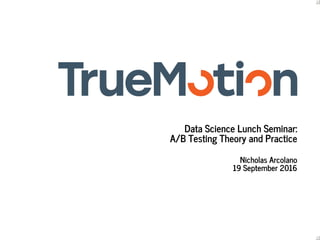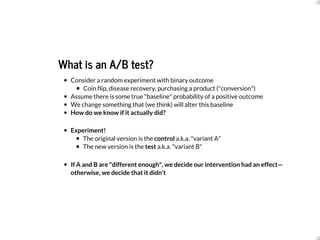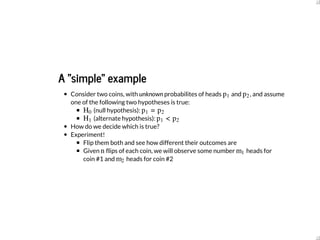Successfully reported this slideshow.

# A/B Testing Theory and Practice (TrueMotion Data Science Lunch Seminar)×

1 of 30
1 of 30

# A/B Testing Theory and Practice (TrueMotion Data Science Lunch Seminar)

TrueMotion Data Science Lunch Seminar for September 19, 2016, wherein we discuss the theory behind A/B testing and some best practices for its real-world application.

TrueMotion Data Science Lunch Seminar for September 19, 2016, wherein we discuss the theory behind A/B testing and some best practices for its real-world application.

## More Related Content

### A/B Testing Theory and Practice (TrueMotion Data Science Lunch Seminar)

1. 1. Data Science Lunch Seminar: A/B Testing Theory and Practice Nicholas Arcolano 19 September 2016
2. 2. What is an A/B test? Consider a random experiment with binary outcome Coin flip, disease recovery, purchasing a product ("conversion") Assume there is some true "baseline" probability of a positive outcome We change something that (we think) will alter this baseline How do we know if it actually did? Experiment! The original version is the control a.k.a. "variant A" The new version is the test a.k.a. "variant B" If A and B are "different enough", we decide our intervention had an effect— otherwise, we decide that it didn't
3. 3. A "simple" example Consider two coins, with unknown probabilites of heads and , and assume one of the following two hypotheses is true: (null hypothesis): (alternate hypothesis): How do we decide which is true? Experiment! Flip them both and see how different their outcomes are Given flips of each coin, we will observe some number heads for coin #1 and heads for coin #2 p1 p2 H0 =p1 p2 H1 <p1 p2 n m1 m2
4. 4. If we knew both distributions, we could just do the optimal thing prescribed by classical binary hypothesis testing—but this would require knowing and Instead, we need some other statistical test that will take , , and and give us a number we can threshold to make a decision p1 p2 n m1 m2
5. 5. A review of statistical tests, errors, and power Basic approach to statistical testing: Determine a test statistic: random variable that depends on , , and Want a statistic whose distribution given the null hypothesis is computable (exactly or approximately) If the data we observe puts us in the tails of the distribution, we say that is too unlikely and "reject the null hypothesis" (choose ) -value: tail probability of the sampling distribution given the null hypothesis is true ( -value too small, reject the null) n m1 m2 H0 H1 p p
6. 6. Often summarize the data as a 2 x 2 contingency table Heads Tails Row totals Coin #1 Coin #2 Column totals Statistical test takes this table and produces a -value, which we then threshold (e.g. ) m1 n − m1 n m2 n − m2 n +m1 m2 2n − −m1 m2 2n p p < 0.05
7. 7. Types of errors Four potential outcomes of the test: is true, choose : true positive (correct detection) is true, choose : true negative is true, choose : false positive (Type I error) is true, choose : false negative (Type II error) H1 H1 H0 H0 H0 H1 H1 H0
8. 8. Power and false positive rate Denote the probabilies of false positives and false negatives as and Since -value represents the tail probability under the null, rejecting corresponds to false positive rate of (for a one-sided test) Refer to probability of correct detection as the power of the test α β p p < α α Pr (choose | true) = 1 − βH1 H1
9. 9. Relationship to precision and recall Assume we do this test a large number of times, so that observed rates of success/failure represent true probabilities Counts for each possible outcome , , , False alarm rate: Recall (correct detection rate): Precision: TP TN FP FN α = FP FP+TN R = 1 − β = TP TP+FN P = TP TP+FP
10. 10. We also have a prior probability for Traditional hypothesis testing doesn't really take this into account The relationship between , , precision and prior is given by So, for a test with fixed power and false positive rate, precision will scale with the prior probability of H1 π = TP + FN TP + FN + TN + FP α β α = (1 − β) P 1 − P π 1 − π H1
11. 11. Examples of tests Fisher's exact test Observe that under the null, the row and column totals follow a hypergeometric distribution Reject the null if the differences between the row and column totals produces a -value less than the given threshold "Exact test": doesn't need to hold only when is large Typically used when sample sizes are "small" Since distribution can only take on discrete values, can be conservative p n
12. 12. Pearson's chi-squared test Compare the observed frequencies of success and If is true, then the variance of is where The test statistic under the null converges to a distribution Compute the chi-square tail probability of the test statistic, reject the null if it exceeds the threshold /nm1 /nm2 H0 /n − /nm1 m2 =σ2 2 (1 − )π̂ π̂ n =π̂ +m1 m2 2n =z2 ( /n − /n)m1 m2 2 σ2 χ2
13. 13. Back to our example Recall: (null hypothesis): (alternate hypothesis): Assume we get to flip each coin times, and let's look at some examples for each hypothesis H0 =p1 p2 H1 <p1 p2 n = 100
14. 14. Case #1: Alternate hypothesis is true In : n = 100 p1 = 0.40 p2 = 0.60 # Compute distributions x = np.arange(0, n+1) pmf1 = stats.binom.pmf(x, n, p1) pmf2 = stats.binom.pmf(x, n, p2) plot(x, pmf1, pmf2)
15. 15. In : In : # Example outcomes m1, m2 = 40, 60 table = [[m1, n-m1], [m2, n-m2]] chi2, pval, dof, expected = stats.chi2_contingency(table) decision = 'reject H0' if pval < 0.05 else 'accept H0' print('{} ({})'.format(pval, decision)) 0.00720957076474 (reject H 0) m1, m2 = 43, 57 table = [[m1, n-m1], [m2, n-m2]] chi2, pval, dof, expected = stats.chi2_contingency(table) decision = 'reject H0' if pval < 0.05 else 'accept H0' print('{} ({})'.format(pval, decision)) 0.0659920550593 (accept H 0)
16. 16. Case #2: Null hypothesis true In : n = 100 p1 = 0.50 p2 = 0.50 # Compute distributions x = np.arange(0, n+1) pmf1 = stats.binom.pmf(x, n, p1) pmf2 = stats.binom.pmf(x, n, p2) plot(x, pmf1, pmf2)
17. 17. In : In : # Example outcomes m1, m2 = 49, 51 table = [[m1, n-m1], [m2, n-m2]] chi2, pval, dof, expected = stats.chi2_contingency(table) decision = 'reject H0' if pval < 0.05 else 'accept H0' print('{} ({})'.format(pval, decision)) 0.887537083982 (accept H 0) # Example outcomes m1, m2 = 42, 58 table = [[m1, n-m1], [m2, n-m2]] chi2, pval, dof, expected = stats.chi2_contingency(table) decision = 'reject H0' if pval < 0.05 else 'accept H0' print('{} ({})'.format(pval, decision)) 0.0338948535247 (reject H 0)
18. 18. Sample size calculation Often what we really want to know is: how many flips to we need to reach a certain level of confidence that we are really observing a difference?
19. 19. Factors affecting required sample size Baseline probability : how often does anything interesting happen? Minimum observable difference that we want to be able to detect between and Desired power of the test: if there is a real difference, how likely do we want to be to observe it? Desired false positive rate of the test So in practice, if we have a good guess at and the minimum that we can accept detecting, we can estimate a minimum p1 p2 p1 p1 p2 n
20. 20. Casagrande et al (1978) Approximate formula gives the desired sample size as a function of , , , and : where is a "correction factor" given by with and where denotes the standard normal quantile function, i.e. is location of the -th quantile for n p1 p2 α β n = A ⎡ ⎣ ⎢ ⎢ ⎢ 1 + 1 + 4( − )p1 p2 A ‾ ‾‾‾‾‾‾‾‾‾‾ √ 2( − )p1 p2 ⎤ ⎦ ⎥ ⎥ ⎥ 2 A χ2 A = ,[ + ]z1−α 2 (1 − )p¯ p¯‾ ‾‾‾‾‾‾‾‾√ z1−β (1 − ) + (1 − )p1 p1 p2 p2‾ ‾‾‾‾‾‾‾‾‾‾‾‾‾‾‾‾‾‾‾‾‾√ 2 = ( + )/2p¯ p1 p2 zp = (p)zp Φ−1 p N(0, 1)
21. 21. Example In : p1, p2 = 0.40, 0.60 alpha = 0.05 beta = 0.05 # Evaluate quantile functions p_bar = (p1 + p2)/2.0 za = stats.norm.ppf(1 - alpha/2) # Two-sided test zb = stats.norm.ppf(1 - beta) # Compute correction factor A = (za*np.sqrt(2*p_bar*(1-p_bar)) + zb*np.sqrt(p1*(1-p1) + p2*(1-p2)))**2 # Estimate samples required n = A*(((1 + np.sqrt(1 + 4*(p1-p2)/A))) / (2*(p1-p2)))**2 print n 149.2852619 21
22. 22. A more practical (and scarier) example Assume we have 5.00% conversion on something we care about (e.g. click- through on a purchase page) We introduce a feature that we think will change conversions by 3% (i.e. from 5.00% to 5.15%) We want 95% power and 5% false positive rate
23. 23. In : So, for test and control combined we'll need at least 1.1 million users. p1, p2 = 0.0500, 0.0515 alpha = 0.05 beta = 0.05 # Evaluate quantile functions p_bar = (p1 + p2)/2.0 za = stats.norm.ppf(1 - alpha/2) # Two-sided test zb = stats.norm.ppf(1 - beta) # Compute correction factor A = (za*np.sqrt(2*p_bar*(1-p_bar)) + zb*np.sqrt(p1*(1-p1) + p2*(1-p2)))**2 # Estimate samples required n = A*(((1 + np.sqrt(1 + 4*(p1-p2)/A))) / (2*(p1-p2)))**2 print n 555118.7638 31 2n =
24. 24. Also, let's verify that this calculation even works... In : n = 555119 n_trials = 10000 # Simulate experimental results when null is true control0 = stats.binom.rvs(n, p1, size=n_trials) test0 = stats.binom.rvs(n, p1, size=n_trials) # Test and control are the sa me tables0 = [[[a, n-a], [b, n-b]] for a, b in zip(control0, test0)] results0 = [stats.chi2_contingency(T) for T in tables0] decisions0 = [x <= alpha for x in results0] # Simulate Experimental results when alternate is true control1 = stats.binom.rvs(n, p1, size=n_trials) test1 = stats.binom.rvs(n, p2, size=n_trials) # Test and control are differ ent tables1 = [[[a, n-a], [b, n-b]] for a, b in zip(control1, test1)] results1 = [stats.chi2_contingency(T) for T in tables1] decisions1 = [x <= alpha for x in results1] # Compute false alarm and correct detection rates alpha_est = sum(decisions0)/float(n_trials) power_est = sum(decisions1)/float(n_trials) print('Theoretical false alarm rate = {:0.4f}, '.format(alpha) + 'empirical false alarm rate = {:0.4f}'.format(alpha_est)) print('Theoretical power = {:0.4f}, '.format(1 - beta) + 'empirical power = {:0.4f}'.format(power_est)) Theoretical false alarm rate = 0.0500, empirical false alarm rate = 0.04 82 Theoretical power = 0.9500, empirical power = 0.9466
25. 25. What if n is too big? The main things influencing are How extreme is—very rare successes make it hard to reach significance The difference between and —small differences are much harder to measure What can we do if is too big to handle? Typically we won't mess with and too much So, our only options are to adjust what we expect to get for and (i.e. change our minimum measurable effect) Or, we can try to increase by measuring something that is more common (e.g. clicks instead of purchases) n p1 p1 p2 n α β p1 p2 p1
26. 26. Practical issues with A/B testing Sometimes it's hard to target the right group (e.g. email tests) It's easy to screw them up Unexpected variations between control and test Contamination between tests (test crossover) Randomization issues (e.g. individuals vs groups) People (especially those outside of data science) are tempted to abuse them Multiple testing Searching for false positives
27. 27. Issue of prior probabilities Can we know if a test is a "sure thing" or not? If we did, then should we even be testing it? Overall, you can spend a lot of time and effort, especially if you want to measure small changes in rare phenomena
28. 28. Some alternatives to traditional A/B testing Multi-armed bandit theory Approaches for simultaneous exploration and exploitation Given a set of random experiments I could perform, how do I choose among them (in order and quantity)? Appropriate when you want to "earn while you learn" Good for quickly exploiting short windows of opportunity
29. 29. Sequential testing In traditional testing ("fixed horizon"), we can't keep looking at the data as it comes in and then quit when we're successful, because we will inflate our false positive rate Benjamin and Hochberg (1995) – approach to controlling false discovery rate for sequential measurements Likelihood ratio test that converges to the "true" false discovery rate over time This is what the stats engine is built onOptimizely
30. 30. Not actually testing We don't always need to A/B test Testing requires engineering and data science resources Potential upside (e.g. in terms of saved future effort or mitigation of risk) has to outweight the cost of developing, performing, and analyzing the test